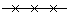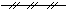# Chemical Engineering - Process Control and Instrumentation

### Exercise :: Process Control and Instrumentation - Section 1

41.

The symbol for "capillary line" in instrumentation diagram is

 A.B.C.D.Explanation:

No answer description available for this question. Let us discuss.

42.

__________ is undesirable in thermocouples used in industries,

 A. Linear relation of emf to temperature B. Corrosion resistance C. Oxidation resistance D. Non-linear relation of emf to temperature

Explanation:

No answer description available for this question. Let us discuss.

43.

Select the correct statement from the following.

 A. The frequency response of a pure capacity process is unbounded. B. The phase lag of a pure time delay system decreases with increasing frequency. C. The amplitude ratio of a pure capacity process is inversely proportional to frequency. D. The amplitude ratio of a pure time delay system increases with frequency.

Explanation:

No answer description available for this question. Let us discuss.

44.

For an input forcing function, X(t) = 2t2, the Laplace transform of this function is

 A. 2/s2 B. 4/s2 C. 2/s3 D. 4/s3

Explanation:

No answer description available for this question. Let us discuss.

45.

Continuous shell temperature measurement in a liquid-liquid heat exchanger is done by a

 A. thermocouple B. reistance thermometer C. mercury in glass thermometer D. vapor pressure thermometer